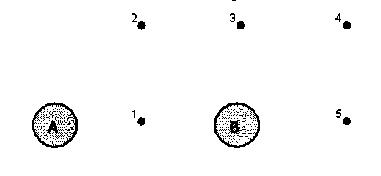## University of California, San Diego Physics 1b - Thermal Physics & Electromagnetism

 H. E. Smith Spring 2000Physics 1B - Tutorial #41. In 5 minutes, timed by your tutor, make a list of all the phenomena that you can think of that are electromagnetic in nature. Think carefully about those phenomena in which the E-M nature is hidden by charge neutrality. The group with the longest (verified!) list in each tutorial will receive gift certificates for free coffee drinks at Espresso Roma. Here's a start
1. atomic structure
2. friction
3. ...

2. Metal spheres A and B, standing on insulated supports, are initially in contact with each other. Sphere C, which has a large negative charge is placed near the other spheres as shown below. While C is nearby, B is moved to the right so that the two spheres are now separated, then C is removed.
• A & B are now charged. Draw a diagram of the charge distribution on each sphere with charge sign indicated. Draw in the E-field lines between the spheres.
• If B is moved toward A, a spark is likely to jump between the spheres before they come in contact. Explain why. Where did the energy (work) for this process originate.3. A and B shown below are stars, of equal mass, in a binary star system. Points 1 - 5 represent small equal test masses. Draw the two forces, FA & FB on each point mass, indicating the magnitude of the force by the length of the vector. Draw the resultant vector at each point with a different colored pen.4. A and B shown above are spherical charges of equal magnitude but opposite sign. Draw the electric fields due to the two charges, EA & EB at each point, indicating the magnitude of the field by the length of the vector. Draw the resultant vector at each point with a different colored pen.

5. Two concentric spherical conducting shells A & B (RA < RB) are charged with charges +Q and -Q respectively. Determine the Elictric Field for a) r < RA, b) RA < r < RB, c) r > rA. Draw a diagram for the E-fields.Physics 1B HomeTutorials

Gene Smith